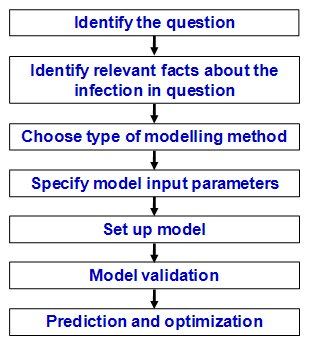# Chapter 2

## How are models set up? An introduction to difference equations

This chapter introduces the key steps for setting models, the main types of models and the key input parameters. Using a model describing the transmission of pandemic influenza as an example, readers are led through the key stages of model development from identifying the question to data collection, developing the model structure and equations, key assumptions, prediction, and validation. The key types of models are discussed and readers are introduced to the methods for writing down difference equations.A summary of the steps in the development and use of a model (after Habbema et al . 1996 )